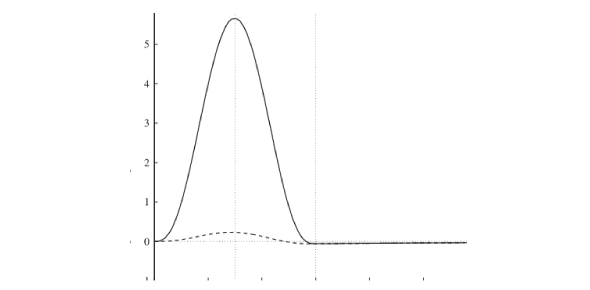What is the best way to calculate the magnitude of a force? - ProProfs Discuss

# What is the best way to calculate the magnitude of a force?Change Image    Delete

This question is part of Distance and Displacement Quiz
Asked by Bilbojaggens, Last updated: Feb 26, 2019

### Request AnswerShare... Answer AnonymouslyAnswer Later Copy Link#### c.Dorothy

C.Dorothy

To measure the force of something, you must first learn the formula for force. It is force which is equal to the mass times the acceleration. The mass should be written in kilograms. If the mass is not written in kilograms, then it should be converted. These are called the International System of Units. Now plug in the mass number and the acceleration number. Then multiply those two numbers together.

If you have the mass and force and need to find the acceleration, then that can be done too. You will divide the force by the mass to get the acceleration. There are differences between weight, force and mass. Weight is only a force when the force is caused by a gravitational object.ReplyQqswadwfe2 ed wsd sqad sqdq dwq dsa qsd qdwdqa qwd q qsd qwdd q dReplyexplain how can it end in a decimal when the distance was not in decimalsReply1.4kmReply#### John Smith

John SmithAnswered on Oct 24, 2016

Calculating the force, or the "resultant vector," requires the ever-useful Pythagorean theorem.ReplySearch for Google imagesSelect a recommended image
Upload from your computerCancelSearch for Google imagesSelect a recommended image
Upload from your computerCancelSearch for Google imagesSelect a recommended image
Upload from your computerCancel